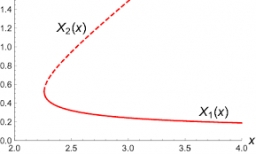# Coefficient 21623

In the equation 2x2 + bx-9 = 0 there is one root x1 = -3 / 2. Determine the second root and the coefficient b

b =  -3
x2 =  -3

### Step-by-step explanation:Did you find an error or inaccuracy? Feel free to write us. Thank you!

Tips for related online calculators
Are you looking for help with calculating roots of a quadratic equation?
Do you have a linear equation or system of equations and looking for its solution? Or do you have a quadratic equation?